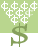Rags to Riches: Answer questions in a quest for fame and fortune.# Finding the slope, y-intercept, and equations of lines.

Using two points on the same line, find the slope and the y-intercept. Then write the equation of the line in slope intercept form. Also, you may be asked to do only one step in this process (find m or find b).This activity was created by a Quia Web subscriber. Learn more about QuiaCreate your own activities